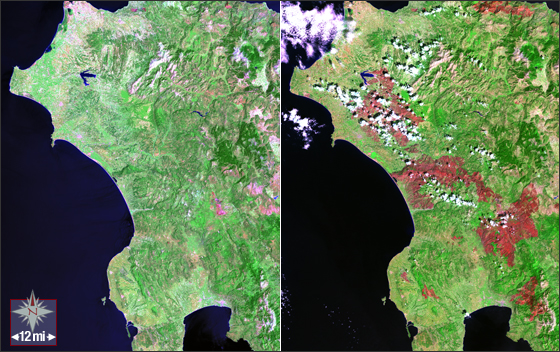## Estimating Biomass Loss from a Large Fire

### Mini Lesson

This is easier to do with the printed Student Page (found under Document Resources). If necessary, you can measure your screen.

1. Using a metric ruler, and the conversion 1 mile = 1.61 kilometers, what is the scale of the image in meters per millimeter?
2. About what is the total area, in square kilometers, of this photo of Greece and its surroundings?
3. About what was the land area, in square kilometers, that was burned?
4. What percentage of the total area was lost to the fires?
5. Suppose that a typical forest in this region contains about 5.0 kilograms of biomass per square meter. How many metric tons of biomass were lost during the fires?Sources:

2. Landsat Math https://landsat.gsfc.nasa.gov/landsat-math-2/
3. Space Math http://spacemath.gsfc.nasa.gov